Question

# a. How many molecules of tetraphosphorus decaoxide are present in 2.96 moles of this compound? 1783x10^23...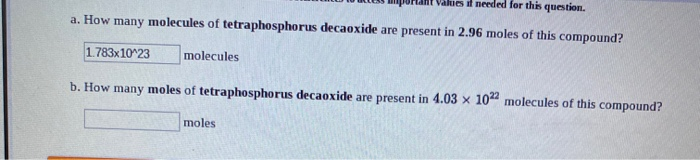a. How many molecules of tetraphosphorus decaoxide are present in 2.96 moles of this compound? 1783x10^23 molecules b. How many moles of tetraphosphorus decaoxide are present in 4.03 x 10" molecules of this compound? moles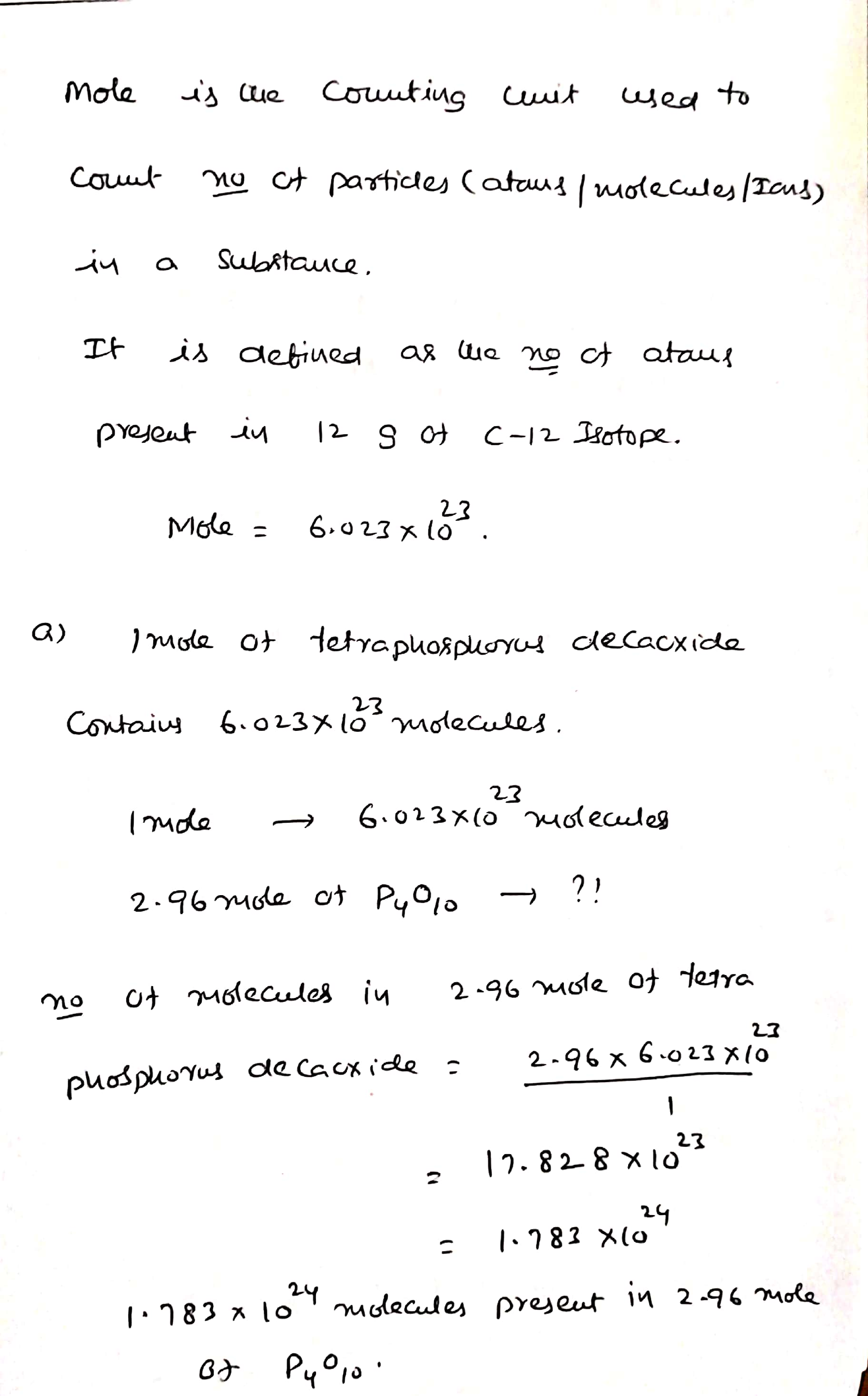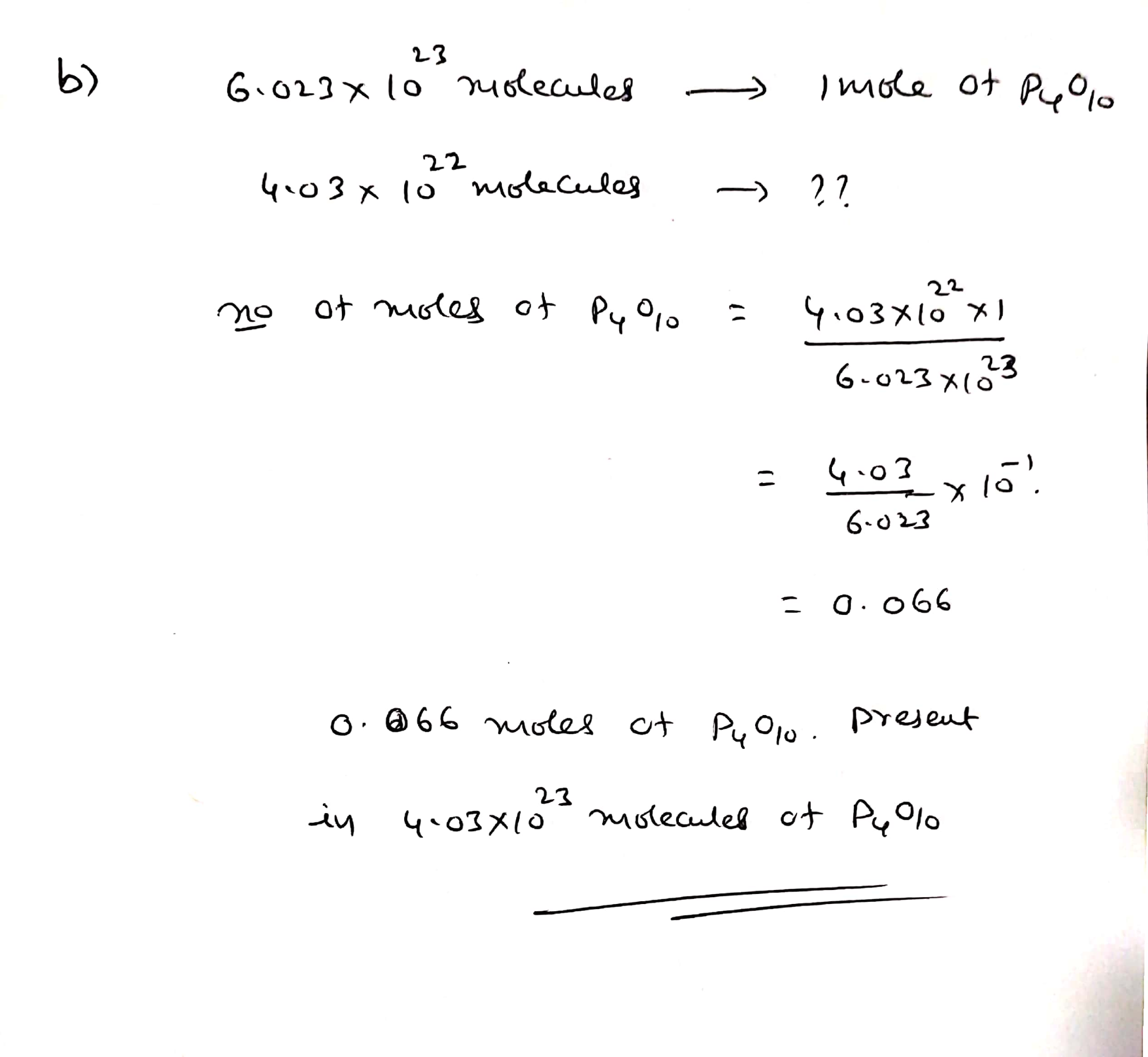#### Earn Coins

Coins can be redeemed for fabulous gifts.

Similar Homework Help Questions
• ### how many molecules of tetraphosphorus decaoxide are present in 4.31 moles of this compound ? and...

how many molecules of tetraphosphorus decaoxide are present in 4.31 moles of this compound ? and how many moles of tetraphosphorus decaoxide are present in 1.07x10^22 molecules of this compound ?

• ### a. How many moles of xenon trioxide are present in 4.95 x 1022 molecules of this...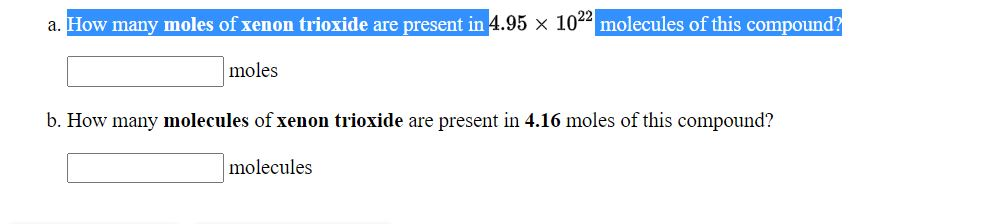a. How many moles of xenon trioxide are present in 4.95 x 1022 molecules of this compound? moles b. How many molecules of xenon trioxide are present in 4.16 moles of this compound? molecules a. How many moles of nitrogen dioxide are present in 2.77 x 1022 molecules of this compound? moles b. How many molecules of nitrogen dioxide are present in 1.31 moles of this compound? molecules a. How many molecules of tetraphosphorus decaoxide are present in 2.05 moles...

• ### Use the References to access important values if needed for this question. How many ATOMS of...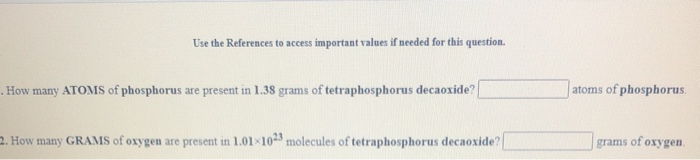Use the References to access important values if needed for this question. How many ATOMS of phosphorus are present in 1.38 grams of tetraphosphorus decaoxide? atoms of phosphorus. 2. How many GRAMS of oxygen are present in 1.01 1023 molecules of tetraphosphorus decaoxide? grams of oxygen days grams 1. How many GRAMS of potassium sulfate are present in 4.96 moles of this compound? moles 2. How many MOLES of potassium sulfate are present in 1.67 grams of this compound?

• ### 1. How many MOLECULES of carbon tetrabromide are present in 2.48 moles of this compound? molecules....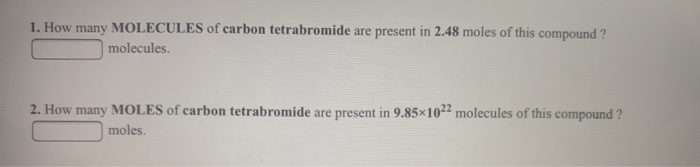1. How many MOLECULES of carbon tetrabromide are present in 2.48 moles of this compound? molecules. 2. How many MOLES of carbon tetrabromide are present in 9.85*1022 molecules of this compound? moles.

• ### How many grams of phosphorus react with 31.2 L of O2 at STP to form tetraphosphorus decaoxide?

How many grams of phosphorus react with 31.2 L of O2 at STP to form tetraphosphorus decaoxide?4sf

• ### locs are present in a sample of HCl with a mass of 3.65g? 4. How many...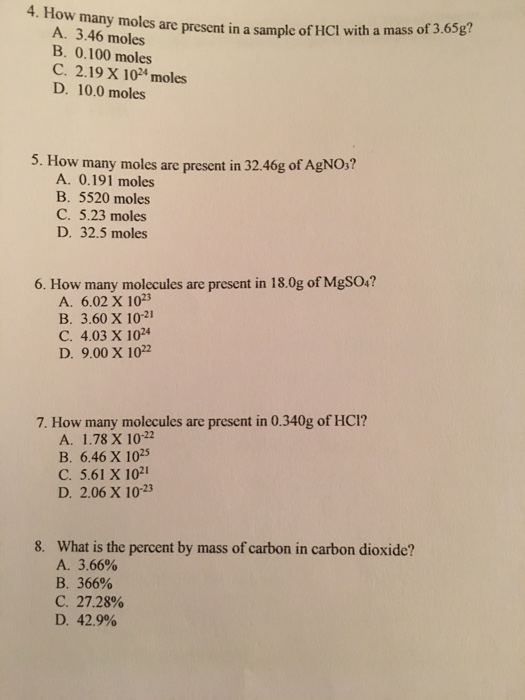locs are present in a sample of HCl with a mass of 3.65g? 4. How many moles are present in a sa A. 3.46 moles B. 0.100 moles C. 2.19 X 1024 moles D. 10.0 moles 5. How many moles are present in 32.46g of AgNO3? A. 0.191 moles B. 5520 moles C. 5.23 moles D. 32.5 moles 6. How many molecules are present in 18.0g of MgSO4? A. 6.02 X 1023 B. 3.60 X 10-21 C. 4.03 X 1024...

• ### How many moles are present if you have 8.75 times 10^23 molecules of CH_4? (include only...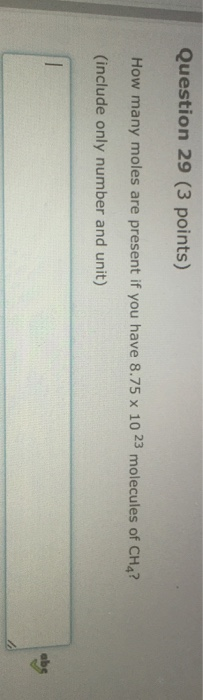How many moles are present if you have 8.75 times 10^23 molecules of CH_4? (include only number and unit)

• ### explanation please 8) How many molecules are present in 4.25 mol of CC14? A) 9.26 x...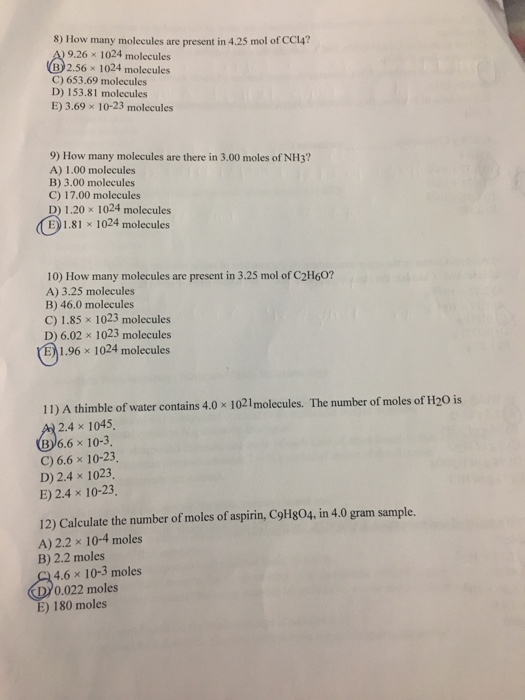explanation please 8) How many molecules are present in 4.25 mol of CC14? A) 9.26 x 1024 molecules (B)2.56 x 1024 molecules C) 653.69 molecules D) 153.81 molecules E) 3.69 x 10-23 molecules 9) How many molecules are there in 3.00 moles of NH3? A) 1.00 molecules B) 3.00 molecules C) 17.00 molecules D) 1.20 x 1024 molecules E1.81x 1024 molecules 10) How many molecules are present in 3.25 mol of C2H60? A) 3.25 molecules B) 46.0 molecules C) 1.85...

• ### a. How many grams of sodium phosphate, Na3PO4, are present in 2.23 moles of this compound?...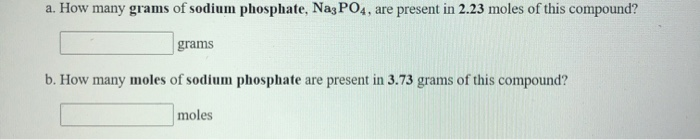a. How many grams of sodium phosphate, Na3PO4, are present in 2.23 moles of this compound? grams b. How many moles of sodium phosphate are present in 3.73 grams of this compound? moles a. How many moles of sulfur hexafluoride, SF6, are present in 2.93 grams of this compound? moles b. How many grams of sulfur hexafluoride are present in 3.30 moles of this compound? grams

• ### 1. How many ATOMS of carbon are present in 4.23 moles of carbon dioxide ? ____atoms...

1. How many ATOMS of carbon are present in 4.23 moles of carbon dioxide ? ____atoms of carbon. 2. How many MOLES of oxygen are present in 3.29×1022 molecules of carbon dioxide ? _____moles of oxygen. 3. How many MOLECULES of carbon tetrabromide are present in 2.73 moles of this compound ? ____molecules. 4. 2. How many MOLES of carbon tetrabromide are present in 9.20×1022 molecules of this compound ? _____moles.